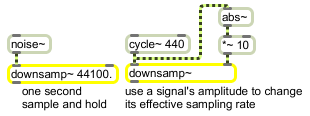downsamp~

Downsample a signal

Description

downsamp~ samples and holds a signal received in the left inlet at a rate set by an argument to the object of the value received in the right inlet, expressed in samples. No interpolation of the output is performed.

Arguments

Name Type Opt Description
downsampled-rate int or float opt Sets the sample rate.

Messages

 int downsampled-rate [int] In right inlet: Sets the sample rate used to downsample the input signal. You can specify the number of samples with floating-point values, but the downsamp~ object will sample the input at most as frequently as the current sampling rate. float downsampled-rate [float] In right inlet: Sets the sample rate used to downsample the input signal. You can specify the number of samples with floating-point values, but the downsamp~ object will sample the input at most as frequently as the current sampling rate. signal In left inlet: A signal to be downsampled. In right inlet: The rate, in samples, at which the incoming signal is to be downsampled.

Output

signal: The input signal, resampled at the rate set by argument or by the value received in the right inlet.

ExamplesSample and hold every n samples